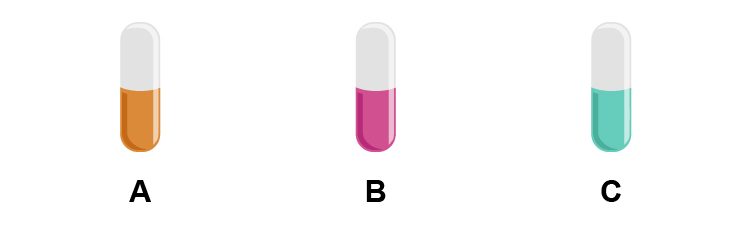# Dice game of probability (Just for fun)

(This will never be in an exam but adds fun to maths and probability and teaches you the language and the strange nature of probability. Teachers should try this in class for fun)

Look at these unusual dice: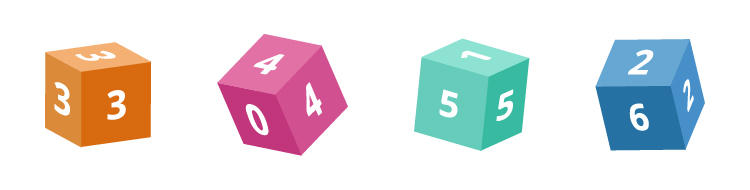We will open each up.

The first is: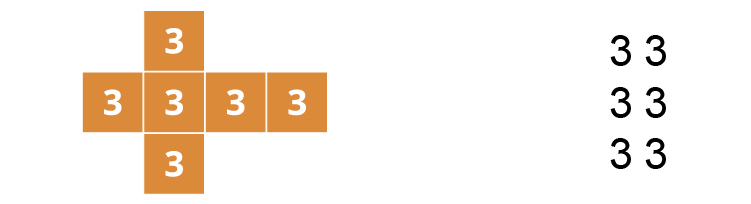The second is: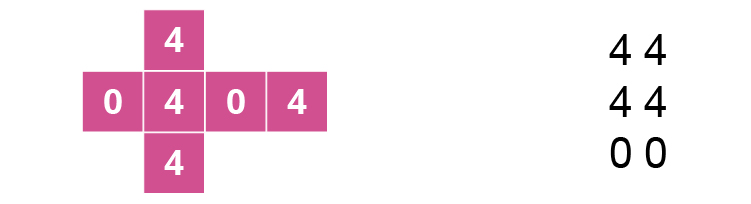The third is: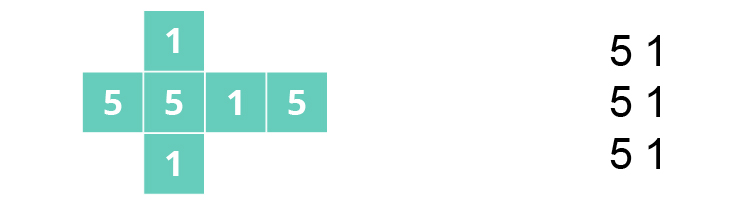The fourth is: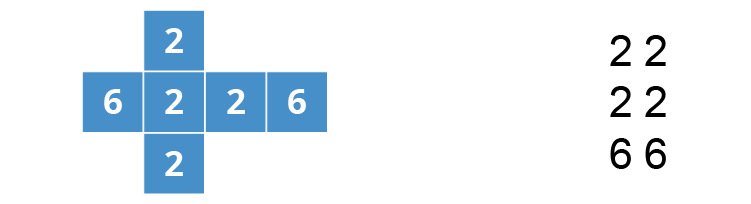If we rank the dice as which is stronger, which is weaker by the definition:

"one die is stronger than the other die if that die has a higher probability of winning against the other die"

which means it wins more frequently.

Conversely

"One die is weaker than the other die if that die has a lower probability of winning against the other die."

Which means it loses more often.

Example 1

Which is the stronger dice?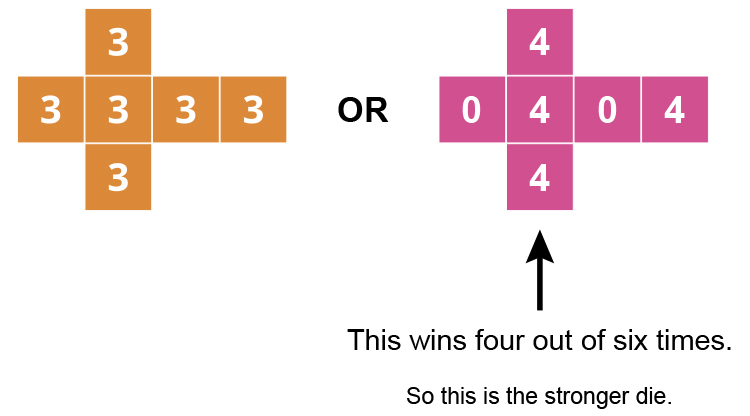So the totem pole ranking would be: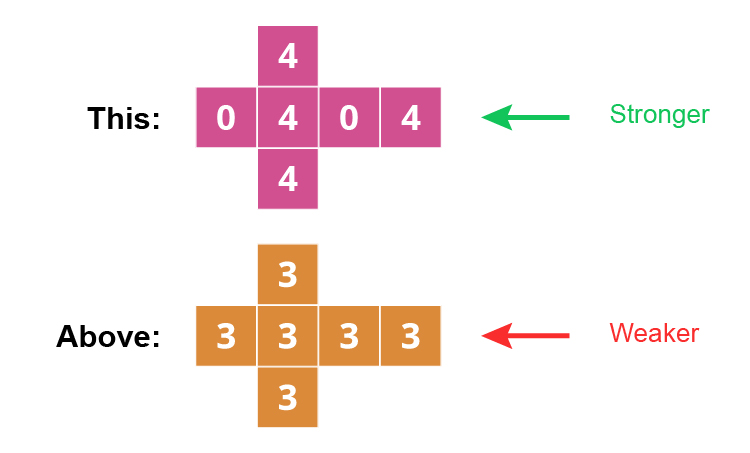Example 2

Which is the stronger dice?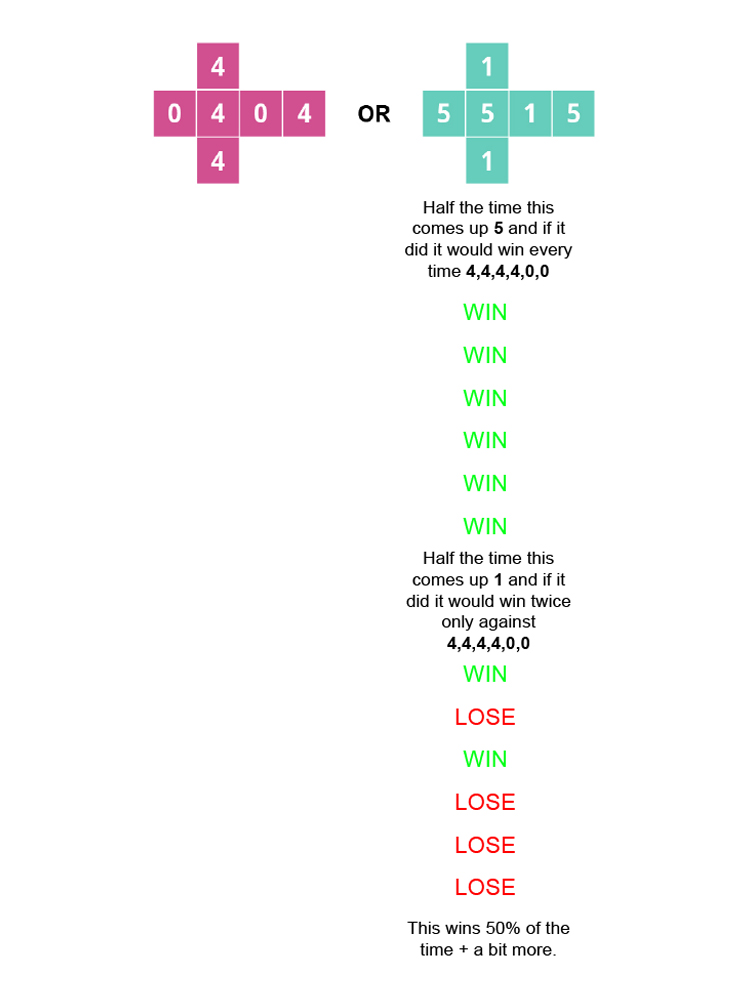So the totem pole ranking would be: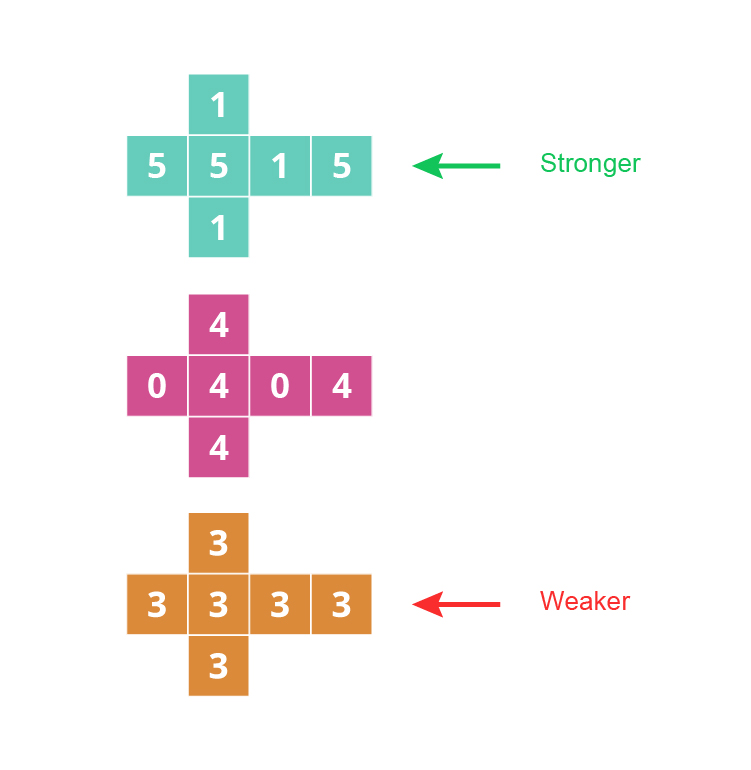Example 3

Which is the stronger dice?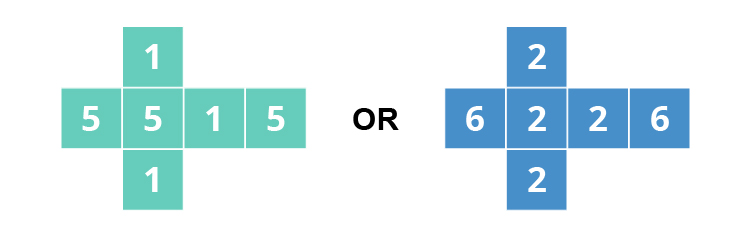Half the time this comes up 1 and if it did it
would lose every time against 6, 6, 2, 2, 2, 2

LOSE

LOSE

LOSE

LOSE

LOSE

LOSE

Half the time this comes up 5 and if it did it
would lose twice against 6, 6, 2, 2, 2, 2

WIN

LOSE

WIN

LOSE

WIN

WIN

This loses 50% of the time + a bit more loss

So this is a weaker die

So the totem pole ranking would be: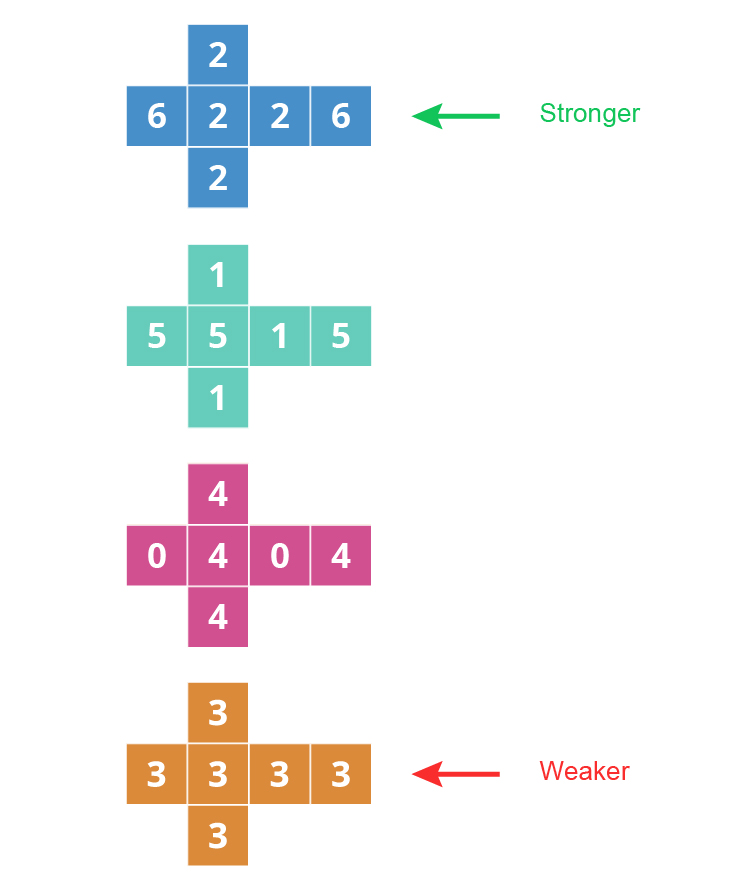Example 4

Now lets compare the two ends. Which is stronger?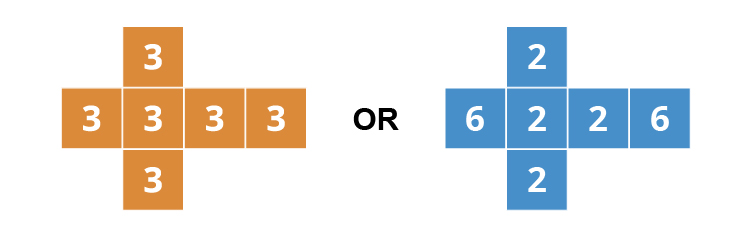You would think this would be the 6, 2, 2, 2, 2, 6 because of the above rankings but

3, 3, 3, 3, 3, 3 wins four out of six times. So this is the stronger die.

So the new totem pole ranking would be: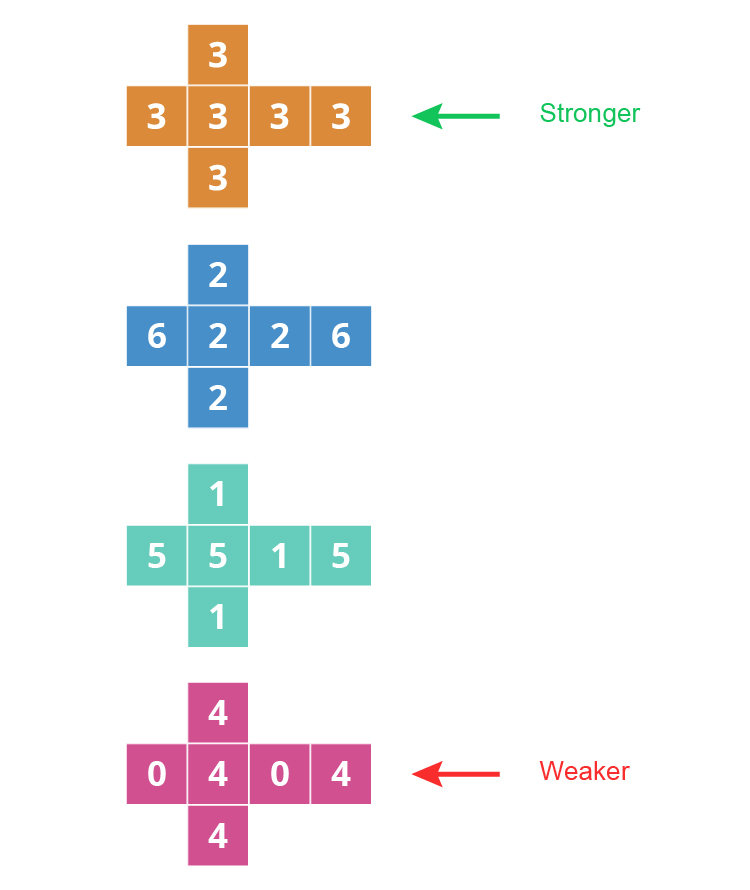Example 5

If we do this again and compare the two ends we are comparing: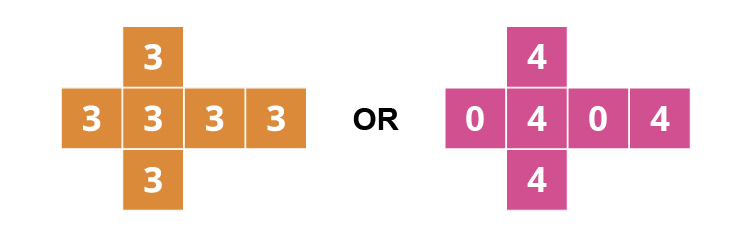AND SO THE CYCLE CONTINUES

This is important for people to know because if you compare 3 drugs: